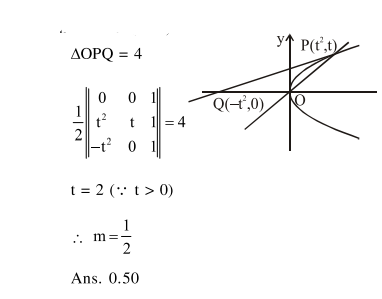# Let a line y=m x(m>0) intersect the parabola,

Question:

Let a line $y=m x(m>0)$ intersect the parabola, $y^{2}=x$ at a point $P$, other than the origin. Let the tangent to it at $P$ meet the $x$-axis at the point Q. If area $(\triangle \mathrm{OPQ})=4 \mathrm{sq}$. units, then $\mathrm{m}$ is equal to_________.

Solution: